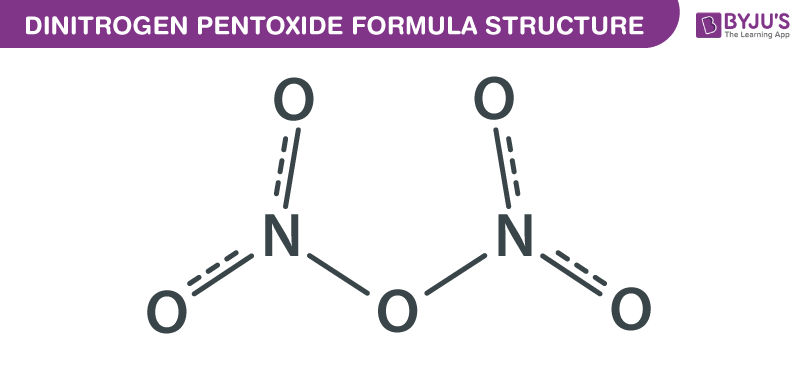# Dinitrogen Pentoxide Formula

Dinitrogen Pentoxide formula, also known as Nitrogen pentoxide formula or Nitroether formula is explained in this article. This salt consists of cations and anions. Linear nitronium ion (NO2+) is the cation and the planar nitrate (NO3) ion is the anion. The chemical or molecular formula of Dinitrogen Pentoxide is N2O5.

It occurs as a colourless to white crystalline solid that sublimes slightly above the room temperature. N2O5 slowly decomposes at room temperature into nitrogen dioxide and oxygen. It is soluble in water and chloroform. In the year 1840, Deville was the first to report Dinitrogen Pentoxide. It can be synthesized by dehydrating nitric acid with phosphorus V oxide. It is widely used as a reagent to introduce nitrogen dioxide functionality.

## Dinitrogen Pentoxide Formula Structure## Properties Of Dinitrogen Pentoxide Formula

 Chemical formula of Dinitrogen Pentoxide N2O5 Molecular weight of Dinitrogen Pentoxide 108.01 g/mol Density of Dinitrogen Pentoxide 1.642 g/cm3 Boiling point of Dinitrogen Pentoxide 47 °C Melting point of Dinitrogen Pentoxide 41°C

It is a strong oxidizer and can form explosive mixtures with ammonium salts and organic compounds. When Nitrogen pentoxide decomposes it produces highly toxic nitrogen dioxide gas.